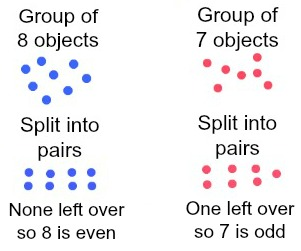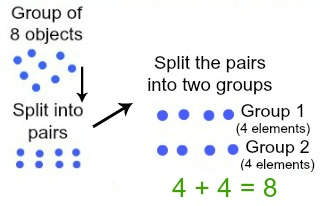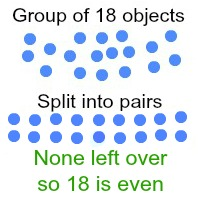# Even Number Pairing & Equations

Instructor: Laura Pennington

Laura received her Master's degree in Pure Mathematics from Michigan State University, and her Bachelor's degree in Mathematics from Grand Valley State University. She has 20 years of experience teaching collegiate mathematics at various institutions.

Integers can be even or odd. This lesson will discuss the concepts of even pairings and even number equations, which will lead to the technical definition of even numbers and allow us to determine if a given number is even or odd.

## Even Number Pairings

Suppose your teacher tells everyone in your class to pair up with one other student. After you all do so, all students are paired, and no one is left without a partner.

Believe it or not, you've just performed a mathematical feat! By pairing off, you've determined that there is an even number of pupils in your class! This is called an even pairing.

An even pairing of a group of objects is the process of pairing the objects in a group in twos. If no objects are left over, we know there is an even number of objects in the group. If there is one object left over, then it is not an even pairing, and there is an odd number of objects in the group.

For example, consider the number 8 and the number 7. If we try to pair a group of eight objects, we will get four sets of pairs with no objects left over. However, if we try to pair a group of seven objects, we will get three sets of pairs with one object leftover.This tells us that 8 is an even number, and 7 is an odd number. Pretty neat, huh?

## Equations of Even Numbers

As we just saw, even numbers can be evenly paired. Suppose we take those pairs of even numbers and split them into two groups, with each group containing one object from each pair. Now we have two groups of equal size. If we add the groups together, we end up with an even number; this tells us that every even number can be written as the sum of two equal numbers.

Let's consider our number 8 again. We said that we can do an even pairing of 8 into four pairs with no objects left over. Now, let's split those four pairs into two groups by putting one object from each pair in one group and the other object from each pair in the other group.Ah-ha! By splitting up the even pairing into two groups, we see that 8 is the sum of two equal numbers, or 8 = 4 + 4.

In general, all even numbers can be written as a sum of two equal numbers. If a is an even number, then

a = b + b

where b is the number of pairs in the even pairing of a.

Notice that a = b + b can also be written as a = 2b. This leads us to the technical definition of an even number, which states that a number a is even if a = 2k, where k is some integer.

This is all pretty exciting! Let's look at an example that can pull all of this information together!

## Example of Even Pairing

Consider the number 18. First, let's determine if it is an even number by seeing if we can do an even pairing with it.Okay, we see that we have an even pairing of the number 18, because we were able to pair up 18 objects into nine pairs without having any objects leftover. Therefore, we know that 18 is an even number!

To unlock this lesson you must be a Study.com Member.

### Register to view this lesson

Are you a student or a teacher?